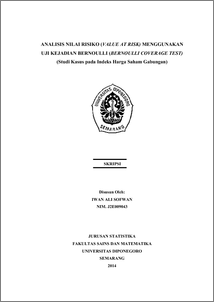# ANALISIS NILAI RISIKO (VALUE AT RISK) MENGGUNAKAN UJI KEJADIAN BERNOULLI (BERNOULLI COVERAGE TEST) (Studi Kasus pada Indeks Harga Saham Gabungan)

SOFWAN, IWAN ALI (2014) ANALISIS NILAI RISIKO (VALUE AT RISK) MENGGUNAKAN UJI KEJADIAN BERNOULLI (BERNOULLI COVERAGE TEST) (Studi Kasus pada Indeks Harga Saham Gabungan). Undergraduate thesis, FSM Undip.Preview
PDF
987Kb

## Abstract

Risk management is a systematic procedure to decrease the risk of an asset. Risk must be calculated in order to determine the best strategy in investing. Value at Risk (VaR) is a measure of risk that can be used. VaR measures the worst loss that can be happen in the future at a certain confidence level. There are many method to compute VaR. However, the methods are useful if it can predict future risks accurately. Therefore, the methods should be evaluate with a backtesting procedure. This research analyze the two methods of computing VaR, Historical Simulation and Johnson S_U transformation approach, that estimate the risk of Jakarta Composite Index and backtest the methods use Bernoulli Coverage Test. The result, if using the relative VaR to forecast the risk of Jakarta Composite Index, the historical simulation approach can be used if the expected probability of violation is 0,002≤p_0≤0,02. Whereas the Johnson S_U transformation approach can be used if the expected probability of violation is 0,001≤p_0≤0,01. If using the absolute VaR to forecast the risk of Jakarta Composite Index, the historical simulation approach can be used if the expected probability of violation is 0,002≤p_0≤0,02. Whereas the Johnson S_U transformation approach can be used if the expected probability of violation is 0,005≤p_0≤0,02. Keywords: Risk, Value at Risk, Backtesting, Bernoulli Coverage Test

Item Type: Thesis (Undergraduate) H Social Sciences > HA Statistics Faculty of Science and Mathematics > Department of Statistics 43459 Mr Hasbi Yasin 12 Aug 2014 11:43 12 Aug 2014 12:13

Repository Staff Only: item control page